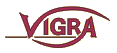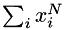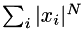Feature AccumulatorsThe namespace vigra::acc provides the function vigra::acc::extractFeatures() along with associated statistics functors and accumulator classes. Together, they provide a framework for efficient compution of a wide variety of statistical features, both globally for an entire image, and locally for each region defined by a label array. Many different statistics can be composed out of a small number of fundamental statistics and suitable modifiers. The user simply selects the desired statistics by means of their tags (see below), and a template meta-program automatically generates an efficient functor that computes exactly those statistics.

The function extractFeatures() scans the data in as few passes as the selected statstics permit (usually one or two passes are sufficient). Statistics are computed by accurate incremental algorithms, whose internal state is maintained by accumulator objects. The state is updated by passing data to the accumulator one sample at a time. Accumulators are grouped within an accumulator chain. Dependencies between accumulators in the accumulator chain are automatically resolved and missing dependencies are inserted. For example, to compute the mean, you also need to count the number of samples. This allows accumulators to offload some of their computations on other accumulators, making the algorithms more efficient. Each accumulator only sees data in the appropriate pass through the data, called its "working pass".

#include <vigra/accumulator.hxx>

Basic statistics:

• PowerSum<N> (computes)
• AbsPowerSum<N> (computes)
• Skewness, UnbiasedSkewness
• Kurtosis, UnbiasedKurtosis
• Minimum, Maximum
• FlatScatterMatrix (flattened upper-triangular part of scatter matrix)
• 4 histogram classes (see below)
• StandardQuantiles (0%, 10%, 25%, 50%, 75%, 90%, 100%)
• ArgMinWeight, ArgMaxWeight (store data or coordinate where weight assumes its minimal or maximal value)
• CoordinateSystem (identity matrix of appropriate size)

Modifiers: (S is the statistc to be modified)

• Normalization  DivideByCount S/Count RootDivideByCount sqrt( S/Count ) DivideUnbiased S/(Count-1) RootDivideUnbiased sqrt( S/(Count-1) )
• Data preparation:  Central substract mean before computing S Principal project onto PCA eigenvectors Whitened scale to unit variance after PCA Coord compute S from pixel coordinates rather than from pixel values Weighted compute weighted version of S Global compute S globally rather than per region (per region is default if labels are given)

Aliases for many important features are implemented (mainly as typedef FullName Alias). The alias names are equivalent to full names. Below are some examples for supported alias names. A full list of all available statistics and alias names can be found in the namespace reference vigra::acc. These examples also show how to compose statistics from the fundamental statistics and modifiers:

Alias Full Name
Count PowerSum<0>
Sum PowerSum<1>
SumOfSquares PowerSum<2>
Mean DivideByCount<PowerSum<1>>
RootMeanSquares   RootDivideByCount<PowerSum<2>>
Moment<N> DivideByCount<PowerSum<N>>
Variance DivideByCount<Central<PowerSum<2>>>
StdDev RootDivideByCount<Central<PowerSum<2>>>
Covariance DivideByCount<FlatScatterMatrix>
RegionCenter Coord<Mean>
CenterOfMass Weighted<Coord<Mean>>

There are a few rules for composing statistics:

• modifiers can be specified in any order, but are internally transformed to standard order: Global<Weighted<Coord<normalization<data preparation<basic statistic
• only one normalization modifier and one data preparation modifier (Central or Principal or Whitened) is permitted
• Count ignores all modifiers except Global and Weighted
• Sum ignores Central and Principal, because sum would be zero
• ArgMinWeight and ArgMaxWeight are automatically Weighted
Here is an example how to use \ref acc::AccumulatorChain to compute statistics. (To use Weighted<> or Coord<> modifiers, see below):

#include <vigra/multi_array.hxx>
#include <vigra/impex.hxx>
#include <vigra/accumulator.hxx>
using namespace vigra::acc;
typedef double DataType;
int size = 1000;
vigra::MultiArray<2, DataType> data(vigra::Shape2(size, size));
a;
std::cout << "passes required: " << a.passesRequired() << std::endl;
extractFeatures(data.begin(), data.end(), a);
std::cout << "Mean: " << get<Mean>(a) << std::endl;
std::cout << "Variance: " << get<Variance>(a) << std::endl;

The acc::AccumulatorChain object contains the selected statistics and their dependencies. Statistics have to be wrapped with acc::Select. The statistics are computed with the acc::extractFeatures function and the statistics can be accessed with acc::get .

Rules and notes:

• order of statistics in Select<> is arbitrary
• up to 20 statistics in Select<>, but Select<> can be nested
• dependencies are automatically inserted
• duplicates are automatically removed
• extractFeatures() does as many passes through the data as necessary
• each accumulator only sees data in the appropriate pass (its "working pass")

The Accumulators can also be used with vector-valued data (vigra::RGBValue, vigra::TinyVector, vigra::MultiArray or vigra::MultiArrayView):

typedef vigra::RGBValue<double> DataType;
AccumulatorChain<DataType, Select<...> > a;
...

To compute weighted statistics (Weighted<>) or statistics over coordinates (Coord<>), the accumulator chain can be used with several coupled arrays, one for the data and another for the weights and/or the labels. "Coupled" means that statistics are computed over the corresponding elements of the involved arrays. This is internally done by means of CoupledScanOrderIterator and vigra::CoupledHandle which provide simultaneous access to several arrays (e.g. weight and data) and corresponding coordinates. The types of the coupled arrays are best specified by means of the helper class vigra::CoupledArrays :

AccumulatorChain<CoupledArrays<3, RGBValue<unsigned char>, double>,
Select<...> > a;

This works likewise for label images which are needed for region statistics (see below). The indxx of the array holding data, weights, or labels respectively can be specified inside the Select wrapper. These index specifiers are: (INDEX is of type int)

• DataArg<INDEX>: data are in array 'INDEX' (default INDEX=1)
• LabelArg<INDEX>: labels are in array 'INDEX' (default INDEX=2)
• WeightArg<INDEX>: weights are in array 'INDEX' (default INDEX=rightmost index)

Pixel coordinates are always at index 0. To collect statistics, you simply pass all arrays to the extractFeatures() function:

using namespace vigra::acc;
vigra::MultiArray<3, double> data(...), weights(...);
AccumulatorChain<CoupledArrays<3, double, double>, // two 3D arrays for data and weights
Select<DataArg<1>, WeightArg<2>, // in which array to look (coordinates are always arg 0)
Mean, Variance, //statistics over values
Coord<Mean>, Coord<Variance>, //statistics over coordinates,
Weighted<Mean>, Weighted<Variance>, //weighted values,
Weighted<Coord<Mean> > > > //weighted coordinates.
a;
extractFeatures(data, weights, a);

This even works for a single array, which is useful if you want to combine values with coordinates. For example, to find the location of the minimum element in an array, you interpret the data as weights and select the Coord<ArgMinWeight> statistic (note that the version of extractFeatures() below only works in conjunction with CoupledArrays, despite the fact that there is only one array involved):

using namespace vigra::acc;
Select<WeightArg<1>, // we interprete the data as weights
Coord<ArgMinWeight> > > // and look for the coordinate with minimal weight
a;
extractFeatures(data, a);
std::cout << "minimum is at " << get<Coord<ArgMinWeight> >(a) << std::endl;

To compute region statistics, you use acc::AccumulatorChainArray. Regions are defined by means of a label array whose elements specify the region ID of the corresponding point. Therefore, you will always need at least two arrays here, which are again best specified using the CoupledArrays helper:

using namespace vigra::acc;
Select<DataArg<1>, LabelArg<2>, // in which array to look (coordinates are always arg 0)
Mean, Variance, //per-region statistics over values
Coord<Mean>, Coord<Variance>, //per-region statistics over coordinates
Global<Mean>, Global<Variance> > > //global statistics
a;
a.ignoreLabel(0); //statistics will not be computed for region 0 (e.g. background)
extractFeatures(data, labels, a);
int regionlabel = ...;
std::cout << get<Mean>(a, regionlabel) << std::endl; //get Mean of region with label 'regionlabel'
In some application it will be known only at run-time which statistics have to be computed. An Accumulator with <b>run-time activation</b> is provided by the \ref acc::DynamicAccumulatorChain class. One specifies a set of statistics at compile-time and from this set one can activate the needed statistics at run-time:

using namespace vigra::acc;
activate<Mean>(a); //at run-time
a.activate("Minimum"); //same as activate<Minimum>(a) (alias names are not recognized)
extractFeatures(data.begin(), data.end(), a);
std::cout << "Mean: " << get<Mean>(a) << std::endl; //ok
//std::cout << "Maximum: " << get<Maximum>(a) << std::endl; // run-time error because Maximum not activated

Likewise, for run-time activation of region statistics, use acc::DynamicAccumulatorChainArray.

Accumulator merging (e.g. for parallelization or hierarchical segmentation) is possible for many accumulators:

using namespace vigra::acc;
extractFeatures(data.begin(), data.end(), a); //process entire data set at once
extractFeatures(data.begin(), data.begin()+data.size()/2, a1); //process first half
extractFeatures(data.begin()+data.size()/2, data.end(), a2); //process second half
a1 += a2; // merge: a1 now equals a0 (within numerical tolerances)

Not all statistics can be merged (e.g. Principal usually cannot, except for some important specializations). A statistic can be merged if the "+=" operator is supported (see the documentation of that particular statistic). If the accumulator chain only requires one pass to collect the data, it is also possible to just apply the extractFeatures() function repeatedly:

using namespace vigra::acc;
extractFeatures(data.begin(), data.begin()+data.size()/2, a); // this works because
extractFeatures(data.begin()+data.size()/2, data.end(), a); // all statistics only need pass 1

More care is needed to merge coordinate-based statistics. By default, all coordinate statistics are computed in the local coordinate system of the current region of interest. That is, the upper left corner of the ROI has the coordinate (0, 0) by default. This behavior is not desirable when you want to merge coordinate statistics from different ROIs: then, all accumulators should use the same coordinate system, usually the global system of the entire dataset. This can be achieved by the setCoordinateOffset() function. The following code demonstrates this for the RegionCenter statistic:

using namespace vigra;
using namespace vigra::acc;
MultiArray<2, double> data(width, height);
MultiArray<2, int> labels(width, height);
a1, a2;
// a1 is responsible for the left half of the image. The local coordinate system of this ROI
// happens to be identical to the global coordinate system, so the offset is zero.
Shape2 origin(0,0);
a1.setCoordinateOffset(origin);
extractFeatures(data.subarray(origin, Shape2(width/2, height)),
labels.subarray(origin, Shape2(width/2, height)),
a1);
// a2 is responsible for the right half, so the offset of the local coordinate system is (width/2, 0)
origin = Shape2(width/2, 0);
a2.setCoordinateOffset(origin);
extractFeatures(data.subarray(origin, Shape2(width, height)),
labels.subarray(origin, Shape2(width, height)),
a2);
// since both accumulators worked in the same global coordinate system, we can safely merge them
a1.merge(a2);

When you compute region statistics in ROIs, it is sometimes desirable to use a local region labeling in each ROI. In this way, the labels of each ROI cover a consecutive range of numbers starting with 0. This can save a lot of memory, because AccumulatorChainArray internally uses dense arrays – accumulators will be allocated for all labels from 0 to the maxmimum label, even when many of them are unused. This is avoided by a local labeling. However, this means that label 1 (say) may refer to two different regions in different ROIs. To adjust for this mismatch, you can pass a label mapping to merge() that provides a global label for each label of the accumulator to be merged. Thus, each region on the right hand side will be merged into the left-hand-side accumulator with the given global label. For example, let us assume that the left and right half of the image contain just one region and background. Then, the accumulators of both ROIs have the label 0 (background) and 1 (the region). Upon merging, the region from the right ROI should be given the global label 2, whereas the background should keep its label 0. This is achieved like this:

std::vector<int> labelMapping(2);
labelMapping = 0; // background keeps label 0
labelMapping = 2; // local region 1 becomes global region 2
a1.merge(a2, labelMapping);

Four kinds of histograms are currently implemented:

 IntegerHistogram Data values are equal to bin indices UserRangeHistogram User provides lower and upper bounds for linear range mapping from values to indices. AutoRangeHistogram Range mapping bounds are defiend by minimum and maximum of the data (2 passes needed!) GlobalRangeHistogram Likewise, but use global min/max rather than region min/max as AutoRangeHistogram will
- The number of bins is specified at compile time (as template parameter int BinCount) or at run-time (if BinCount is zero at compile time). In the first case the return type of the accumulator is TinyVector<double, BinCount> (number of bins cannot be changed). In the second case, the return type is MultiArray<1, double> and the number of bins must be set before seeing data (see example below).
- If UserRangeHistogram is used, the bounds for the linear range mapping from values to indices must be set before seeing data (see below).
- Options can be set by passing an instance of HistogramOptions to the accumulator chain (same options for all histograms in the chain) or by directly calling the appropriate member functions of the accumulators.
- Merging is supported if the range mapping of the histograms is the same.
- Histogram accumulators have two members for outliers (left_outliers, right_outliers).

With the StandardQuantiles class, <b>histogram quantiles</b> (0%, 10%, 25%, 50%, 75%, 90%, 100%) are computed from a given histgram using linear interpolation. The return type is TinyVector<double, 7> .


Usage:

using namespace vigra::acc;
typedef double DataType;
typedef UserRangeHistogram<40> SomeHistogram; //binCount set at compile time
typedef UserRangeHistogram<0> SomeHistogram2; // binCount must be set at run-time
typedef AutoRangeHistogram<0> SomeHistogram3;
//set options for all histograms in the accumulator chain:
histogram_opt = histogram_opt.setBinCount(50);
//histogram_opt = histogram_opt.setMinMax(0.1, 0.9); // this would set min/max for all three histograms, but range bounds
// shall be set automatically by min/max of data for SomeHistogram3
a.setHistogramOptions(histogram_opt);
// set options for a specific histogram in the accumulator chain:
getAccumulator<SomeHistogram>(a).setMinMax(0.1, 0.9); // number of bins must be set before setting min/max
getAccumulator<SomeHistogram2>(a).setMinMax(0.0, 1.0);
extractFeatures(data.begin(), data.end(), a);
vigra::TinyVector<double, 40> hist = get<SomeHistogram>(a);
vigra::MultiArray<1, double> hist2 = get<SomeHistogram2>(a);
vigra::TinyVector<double, 7> quant = get<Quantiles3>(a);
double right_outliers = getAccumulator<SomeHistogram>(a).right_outliers;

 © Ullrich Köthe (ullrich.koethe@iwr.uni-heidelberg.de) Heidelberg Collaboratory for Image Processing, University of Heidelberg, Germany html generated using doxygen and Python vigra 1.11.1 (Fri May 19 2017)